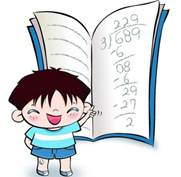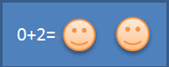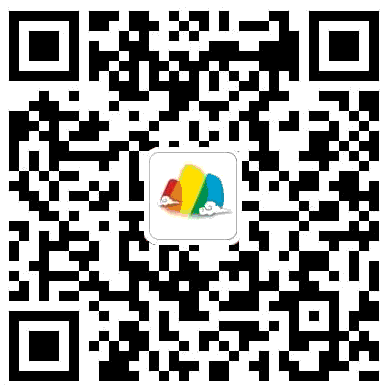# 循序渐进学运算 （摘自《你的孩子也能成为爱因斯坦》）

2015-03-26 13:43:00

3183家人与老师应共同引导孩子，尽早系统地接触数学运算。我们从10以内的数字开始学起（即0123456789）。对于年龄较小的孩子，我们建议制作一些小卡片，并在一旁用图形的多少表示数字的含义。当孩子掌握了这10个数字以后，我们就可以接着教加减乘除的四则运算法则了。接下来，教孩子1015间的数字。在进入16以上的数字时，告诉他们数字叠加的逻辑规律：1610617107……

——重复练习。有时候，老师布置给孩子四道加法练习题，本质上大同小异，也许只做两道或一道便足矣。把不同练习穿插进行，提高孩子的兴趣。

——过度学习。

——对运算步骤的解释越详细越好，避免含混不清、模棱两可地一笔带过。0+0=0   1+0=1   2+0=2   3+0=3   4+0=4   5+0=5

0+1=1   1+1=2   2+1=3   3+1=4   4+1=5   5+1=6

0+2=2   1+2=3   2+2=4   3+2=5   4+2=6   5+2=7

0+3=3   1+3=4   2+3=5   3+3=6   4+3=7   5+3=8

0+4=4   1+4=5   2+4=6   3+4=7   4+4=8   5+4=9

0+5=5   1+5=6   2+5=7   3+5=8   4+5=9

0+6=6   1+6=7   2+6=8   3+6=9

0+7=7   1+7=8   2+7=9

0+8=8   1+8=9

0+9=9

6+0=6   7+0=7   8+0=8   9+0=9

6+1=7   7+1=8   8+1=9

6+2=8   7+2=9

6+3=9

1-0=1   2-0=2   3-0=3   4-0=4   5-0=5   6-0=6   7-0=7

1-1=0   2-1=1   3-1=2   4-1=3   5-1=4   6-1=5   7-1=6

2-2=0   3-2=1   4-2=2   5-2=3   6-2=4   7-2=5

3-3=0   4-3=1   5-3=2   6-3=3   7-3=4

4-4=0   5-4=1   6-4=2   7-4=3

5-5=0   6-5=1   7-5=2

6-6=0   7-6=1

7-7=0

8-0=8   9-0=9

8-1=7   9-1=8

8-2=6   9-2=7

8-3=5   9-3=6

8-4=4   9-4=5

8-5=3   9-5=4

8-6=2   9-6=3

8-7=1   9-7=2

8-8=0   9-8=1

9-9=0

——两个相同的数字相加，计算起来更容易。如：

8+8=16  7+7=14

——颠倒顺序便于记忆：

8+7=15  7+8=15

——把大数放在第一个加数的位置，计算起来更容易。如：

8+3=11    3+8=11  更易计算

——遇到上述情况时，脑中要形成条件反射，迅速运行加法交换律。

——当加数多于两个时，为了简化运算，我们可以运用加法结合律，进行组合计算。如：

3+4+7=10+4=14

——当加减法中出现999999这样的数字时，可以用101001000来简化运算，最后在结果上加减1。如：

6748+999=6748+1000-1=7747

6748-999=6748-1000+1=5749

——同样的，遇到898998这类数字也可以这么处理。记得要在结果上加减2

——当遇到整十、整百、整千类的数字时，可以先将数字末尾的零抹去，在返回结果时再将零添上。此外，1乘以任何数等于该数本身，0乘以任何数都得0

3+3+3=3×3

9:3= 9-3

6-3

3-3

，是所有数字末位余下的数字；则分别由整十、整百、整千个数字拼合而成。与之前一样，教这个概念的时候，可以用10个由10个小圆圈组成的组合来加深孩子的印象，同时锻炼孩子的动手能力。《你的孩子也能成为爱因斯坦》教育界泰斗钱理群教授、中科院院士褚君浩教授推荐，畅销欧美的最新脑科学理论，全面提升孩子学习能力蓝宝书！购买链接最后编辑：ppxuan 于 2015-04-07 16:03:36

发表评论
评论通过审核之后才会显示。
分类免费预约体验课